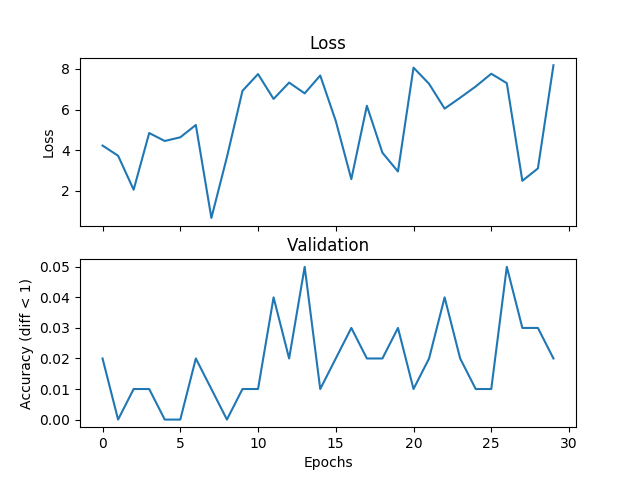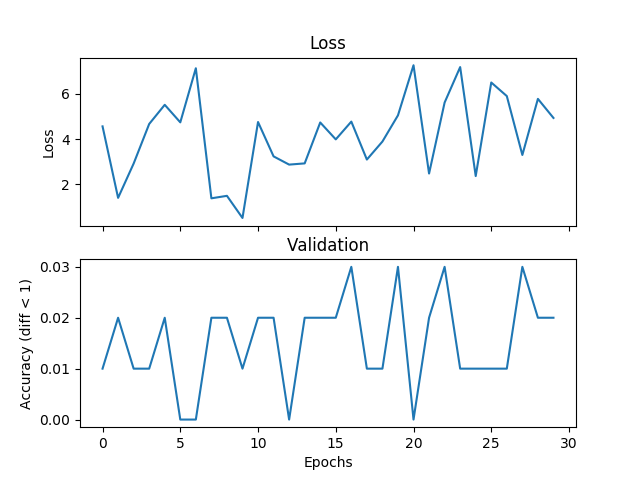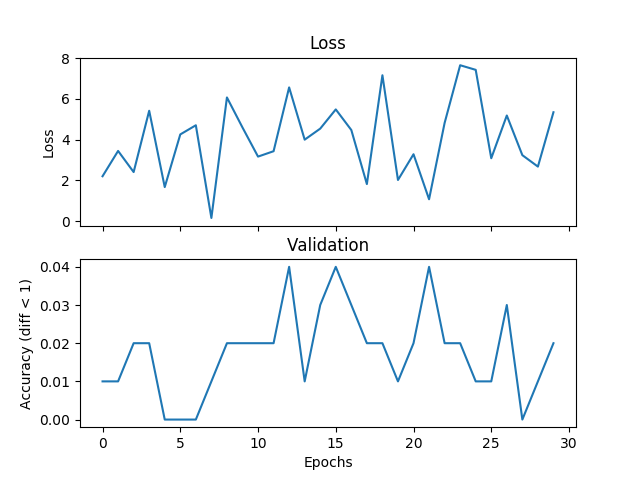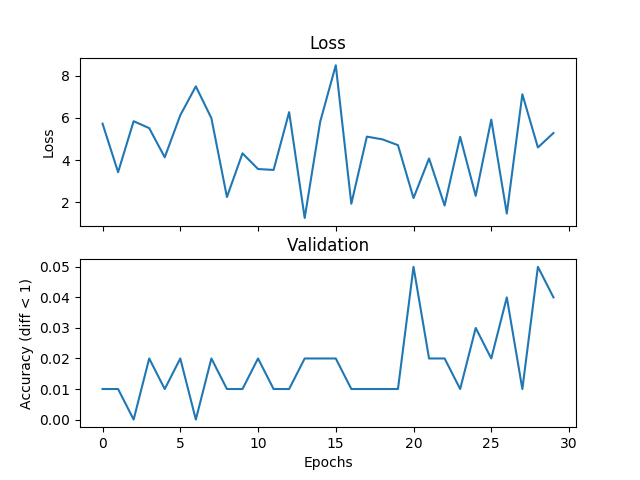# Regression loss functions incorrect

I’m trying a basic averaging example, but the validation and loss don’t match and the network fails to converge if I increase the training time. I’m training a network with 2 hidden layers, each 500 units wide on three integers from the range [0,9] with a learning rate of 1e-1, Adam, batch size of 1, and dropout for 3000 iterations and validate every 100 iterations. If the absolute difference between the label and the hypothesis is less than a threshold, here I set the threshold to 1, I consider that correct. Could someone let me know if this is an issue with the choice of loss function, something wrong with Pytorch, or something I’m doing. Below are some plots:

`val_diff = 1`
`acc_diff = torch.FloatTensor([val_diff]).expand(self.batch_size)`
Loop 100 times to during validation:
`num_correct += torch.sum(torch.abs(val_h - val_y) < acc_diff)`
Append after each validation phase:
`validate.append(num_correct / total_val)`

Here are some examples of the (hypothesis, and labels):

`[...(-0.7043088674545288, 6.0), (-0.15691305696964264, 2.6666667461395264),`     `(0.2827358841896057, 3.3333332538604736)]`

I tried six of the loss functions in the API that are typically used for regression:

torch.nn.L1Loss(size_average=False)torch.nn.L1Loss()torch.nn.MSELoss(size_average=False)torch.nn.MSELoss()torch.nn.SmoothL1Loss(size_average=False)torch.nn.SmoothL1Loss()Thanks.